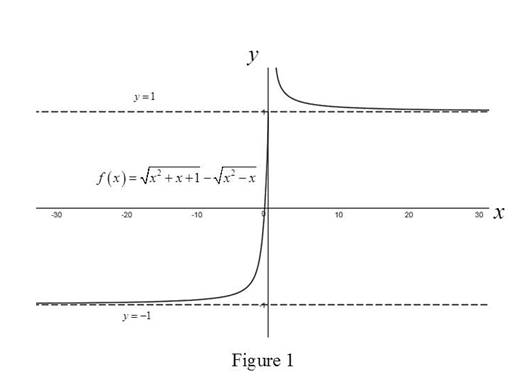# The graph of function f ( x ) = x 2 + x + 1 − x 2 − x and discover the asymptotes.### Single Variable Calculus: Concepts...

4th Edition
James Stewart
Publisher: Cengage Learning
ISBN: 9781337687805### Single Variable Calculus: Concepts...

4th Edition
James Stewart
Publisher: Cengage Learning
ISBN: 9781337687805

#### Solutions

Chapter 2, Problem 20RE
To determine

## To sketch: The graph of function f(x)=x2+x+1−x2−x and discover the asymptotes.

Expert Solution

The horizontal asymptotes of the function f are y=1 and y=1.

### Explanation of Solution

Theorem used:

1. If r>0 is a rational number, then limx1xr=0.

2. If r>0 is a rational number such that xr is defined, then limx1xr=0.

Definition used: Horizontal asymptote:

The line y=L is called a horizontal asymptote of the curve y=f(x) if either limxf(x)=L or limxf(x)=L.

Calculation:

Consider the function f(x)=x2+x+1x2x.

The graph of f(x)=x2+x+1x2x is shown below in Figure 1,From the Figure 1, it is observed that y=1 and y=1 are the horizontal asymptotes to the function f(x)=x2+x+1x2x.

Consider the function f(x)=x2+x+1x2x.

f(x)=x2+x+1x2x=(x2+x+1x2x)1×x2+x+1+x2xx2+x+1+x2x=(x2+x+1x2x)(x2+x+1+x2x)x2+x+1+x2x=(x2+x+1)2(x2x)2x2+x+1+x2x

Simplify the terms of numerator,

f(x)=x2+x+1(x2x)x2+x+1+x2x=2x+1x2+x+1+x2x=2x+1x2(1+1x+1x2)+x2(11x)=x(2+1x)|x|{1+1x+1x2+11x}(|x|=x2)

Case 1: When x>0 implies |x|=x,

f(x)=x(2+1x)x{1+1x+1x2+11x}=(2+1x){1+1x+1x2+11x}

Take limit on the above expression

limxf(x)=limx(2+1x){1+1x+1x2+11x}=limx(2+1x)limx{1+1x+1x2+11x}=limx(2)+limx1x{limx(1+1x+1x2)+limx(11x)}=2+limx1x{(1+limx1x+limx1x2)+1limx1x}

Since r>0, the above mentioned theorem 1 is used to compute the value of the limit function.

limxf(x)=2+01+0+0+10=21+1=1

Thus, the horizontal asymptote to f is y=1.

Case 2: When x<0 implies |x|=x,

f(x)=x(2+1x)x{1+1x+1x2+11x}=(2+1x){1+1x+1x2+11x}

Take limit on the above expression,

limxf(x)=limx(2+1x){1+1x+1x2+11x}=limx(2+1x)limx{1+1x+1x2+11x}=limx(2)+limx1x{limx(1+1x+1x2)+limx(11x)}=2+limx1x{(1+limx1x+limx1x2)+1limx1x}

Since r>0, apply the theorem 2 stated above to compute the value of the limit function.

limxf(x)=2+01+0+0+10=21+1=1

So, the horizontal asymptote to f is y=1.

Thus, it has been verified that y=1 and y=1 are the horizontal asymptotes to the function f(x).

### Have a homework question?

Subscribe to bartleby learn! Ask subject matter experts 30 homework questions each month. Plus, you’ll have access to millions of step-by-step textbook answers!